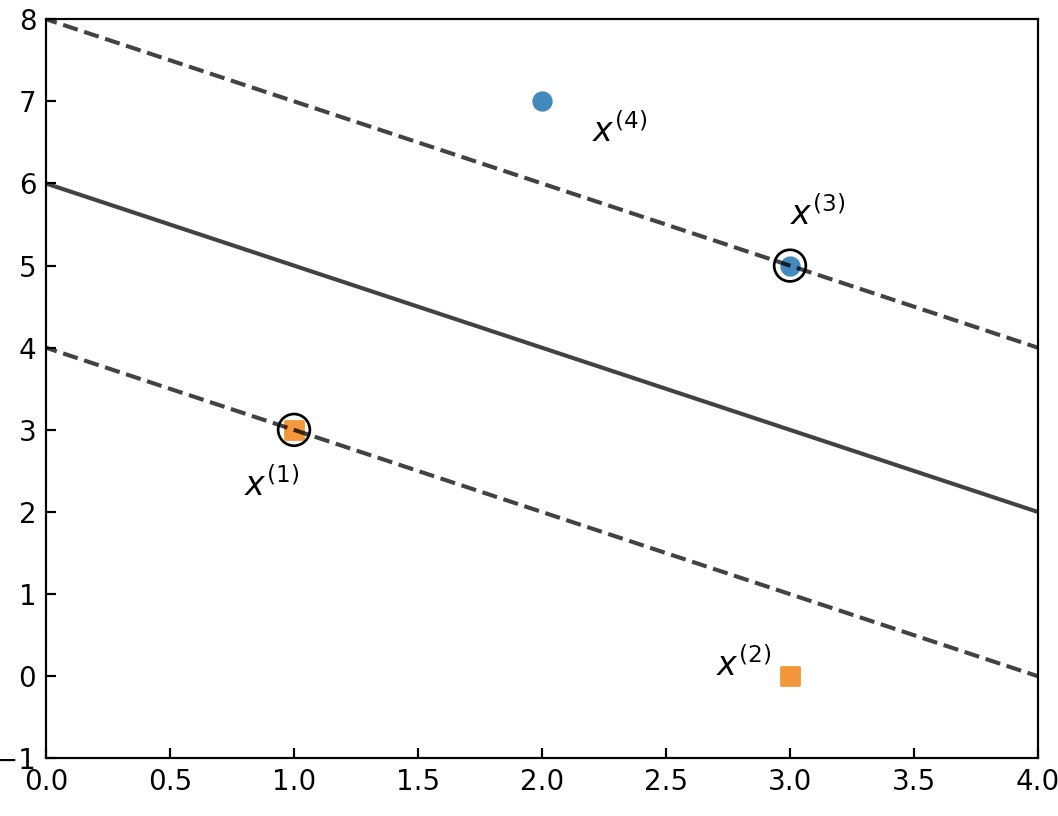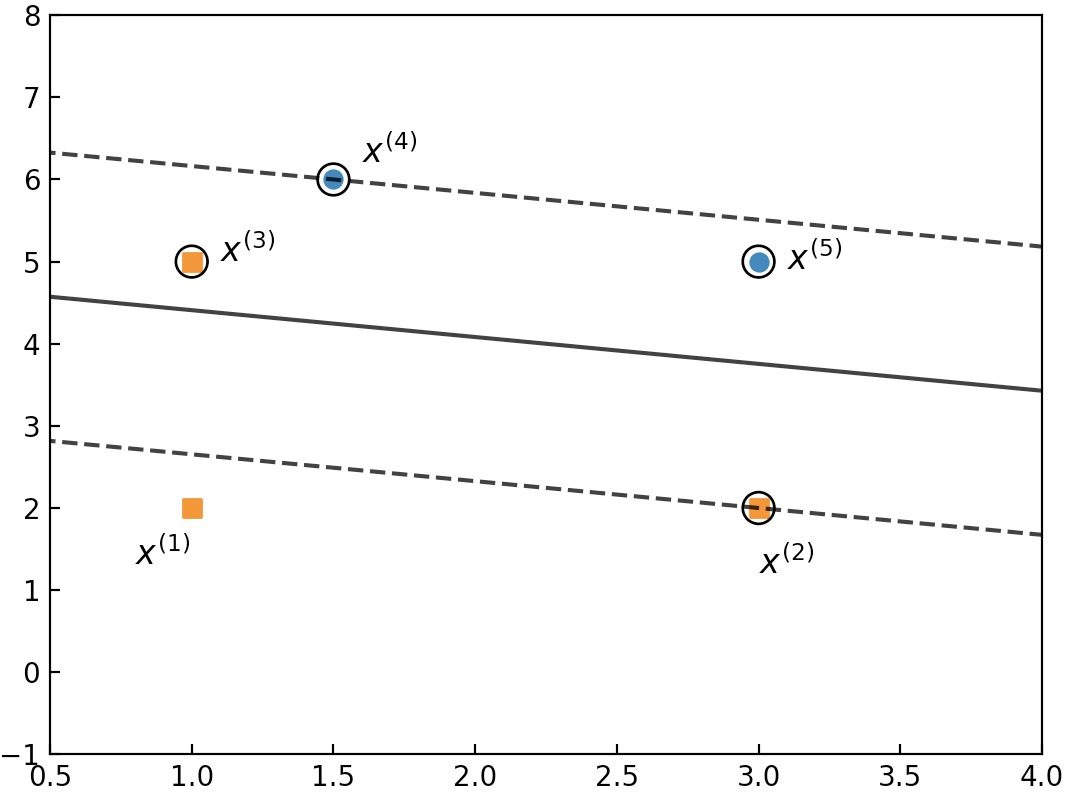### 1 ň╝ĽŔĘÇ

š╗ĆŔ┐çňëŹÚŁóňçáŔŐéňćůň«╣šÜäń╗őš╗Ź´╝îŠłĹń╗ČňĚ▓š╗ĆščąÚüôń║ćŠö»ŠîüňÉĹÚçĆŠť║ŔâîňÉÄšÜäňÄčšÉćŃÇéňÉîŠŚÂ´╝îńŞ║ń║ćŠ▒éŔžúSVMńŞşšÜäšŤ«ŠáçňçŻŠĽ░´╝îšČöŔÇůŔ┐śňťĘňëŹÚŁóńŞĄŔŐéňćůň«╣ńŞşÚÖćš╗şń╗őš╗Źń║ćŠőëŠá╝ŠťŚŠŚąń╣śŠĽ░Š│Ľňĺîň»╣ňüÂŠÇžÚŚ«ÚóśŃÇéŠÄąńŞőŠŁą´╝îňťĘŔ┐Öš»çŠľçšźáńŞşň░ćň╝ÇňžőŠşúň╝Ćń╗őš╗ŹSVMšÜäŠ▒éŔžúŔ┐çšĘőŃÇéňÉîŠŚÂ´╝îńŞ║ń║ćńż┐ń║ÄňÉäńŻŹŔ»╗ŔÇůŠťőňĆőňż¬ň║ĆŠŞÉŔ┐ŤšÜäń║ćŔžúŠĽ┤ńŞ¬Š▒éŔžúŔ┐çšĘő´╝îńŞőÚŁóšČöŔÇůń╝ÜńżŁŠČíňůłňÉÄń╗őš╗ŹšíČÚŚ┤ÚÜöňĺîŔŻ»ÚŚ┤ÚÜöńŞşšŤ«ŠáçňçŻŠĽ░šÜäŠ▒éŔžúŠşąÚ¬ĄŃÇé

### 2 Š×äÚÇášíČÚŚ┤ÚÜöň╣┐ń╣ëŠőëŠá╝ŠťŚŠŚąňçŻŠĽ░

šö▒ňëŹÚŁóňçáš»çŠľçšźášÜäňćůň«╣ňĆ»ščą´╝îSVMšíČÚŚ┤ÚÜöŠťÇš╗łšÜäń╝śňîľšŤ«ŠáçńŞ║

šö▒ŠşĄňĆ»ń╗ąňżŚňł░ň╣┐ń╣ëšÜäŠőëŠá╝ŠťŚŠŚąňçŻŠĽ░ńŞ║

ňůÂńŞş${{\alpha }_{i}}\ge 0$ńŞ║ŠőëŠá╝ŠťŚŠŚąń╣śňşÉ´╝îńŞöňÉîŠŚÂŔ«░

Ŕ┐ŤńŞÇŠşąňĆ»ňżŚňÄčňžőÚŚ«ÚóśšÜäň»╣ňüÂń╝śňîľÚŚ«ÚóśńŞ║

ŠëÇń╗ą´╝îńŞ║ń║ćŠ▒éňżŚň»╣ňüÂÚŚ«ÚóśšÜäŔžú´╝îÚťÇŔŽüŠîëšůžň╝Ć$(4)$ńŞşšÜäÚí║ň║ĆŔ┐ŤŔíîŠ▒éŔžúŃÇé

#### 2.1 ňů│ń║ÄňĆéŠĽ░w,bŠ▒é$\mathcal{L}$šÜäŠ×üň░ĆňÇ╝$W(\alpha )$

ńŞ║ń║ćŠ▒éŔžúň╝Ć$(4)$ńŞşšÜäň»╣ňüÂń╝śňîľÚŚ«Úóś´╝îÚŽľňůłÚťÇŔŽüŠťÇň░Ćňîľň╝Ć$(2)$´╝îňŹ│ňłćňłźň»╣$w,b$Š▒éňüĆň»╝ŠĽ░ň╣Âń╗ĄňůÂńŞ║0Šťë

Ŕ┐ŤńŞÇŠşąŠťë

ňł░ŠşĄńż┐ňżŚňł░ń║ćŠŁâÚçŹ$w$šÜäŔžúŠ×ÉŔíĘŔżżň╝Ć´╝îŔÇîň«âň»╣ń║ÄšÉćŔžúSVMńŞşŠáŞňçŻŠĽ░šÜäňłęšöĘŠťëšŁÇÚçŹŔŽüńŻťšöĘŃÇéŠÄąšŁÇň░ćň╝Ć$(5)(6)$ňŞŽňůąň╝Ć$(2)$ňĆ»ňżŚ

ňîľš«ÇňżŚňł░ň╝Ć$(7)$šÜäňůĚńŻôŠşąÚ¬ĄńŞ║

ň░ćň╝Ć$(5)(6)$ňŞŽňůą$(8)$ňżŚ

ňł░ŠşĄ´╝îńż┐ňżŚňł░ń║ćňĆéŠĽ░$w$šÜäŔžúŠ×Éň╝ĆŃÇé

#### 2.2 ňů│ń║ÄňĆéŠĽ░$\alpha$Š▒é$W(\alpha)$šÜäŠ×üňĄžňÇ╝

šö▒ň╝Ć$(7)$ňĆ»ń╗ąňżŚňç║ňŽéńŞőń╝śňîľÚŚ«Úóś

ń╣őŠëÇń╗ąň╝Ć$(5)$ńŞşŠťÇňÉÄńŞÇńŞ¬šşëň╝Ćń╣čń╝ÜŠłÉńŞ║š║ŽŠŁčŠŁíń╗Â´╝îŠś»ňŤáńŞ║$W(\alpha)$Šś»ÚÇÜŔ┐çň╝Ć$(5)$Š▒éŔžúňżŚňł░Šś»$W(\alpha)$ňşśňťĘšÜäňëŹŠĆÉŃÇé

Ŕ┐ŤńŞÇŠşą´╝î ňüçŔ«ż${{\alpha }^{*}}={{(\alpha _{1}^{*},\alpha _{2}^{*},...,\alpha _{m}^{*})}^{T}}$ńŞ║ň»╣ňüÂń╝śňîľÚŚ«ÚóśšÜäŔžú´╝îÚéúń╣łńŞ║ń║ćńŻ┐ňżŚň»╣ňüÂń╝śňîľÚŚ«ÚóśńŞÄňÄčňžőń╝śňîľÚŚ«ÚóśňÉîŔžúÚťÇŔŽüŠ╗íŔÂ│ňŽéńŞőKKTŠŁíń╗Â

Šá╣ŠŹ«ň╝Ć$(11)$ňĆ»ňżŚ

ń╗Äň╝Ć$(16)$ňĆ»ń╗ąšťőňç║´╝îŔç│ň░ĹňşśňťĘńŞÇńŞ¬$\alpha _{j}^{*}>0$ŃÇéňŤáńŞ║ňŽéŠ×ťŠëÇŠťëšÜä$\alpha_i$ňŁçńŞ║0´╝îÚéúń╣łšö▒ň╝Ć$(16)$ňĆ»ščąŠşĄŠŚÂšÜä$w^{*}$ń╣čńŞ║0´╝îŔÇîŔ┐ÖŠśżšäÂńŞŹŠś»ňÄčňžőń╝śňîľÚŚ«ÚóśšÜäŔžúŃÇé

ňÉîŠŚÂ´╝îšö▒ň╝Ć$(13)$ňĆ»ščą´╝îŔőąňşśňťĘ$\alpha _{j}^{*}>0$´╝îÚéúń╣łň┐ůŠťë

Šá╣ŠŹ«ň╝Ć$(17)$ňĆ»ščą´╝îŠşĄŠŚÂšÜäŠáĚŠťČšé╣${{x}^{(j)}}$Šś»ńŞÇńŞ¬Šö»ŠîüňÉĹÚçĆ´╝îŔÇîŔ┐Öń╣čŠśżšĄ║ňç║šÜäńŞÇńŞ¬ÚçŹŔŽüŠÇžŔ┤ĘŠś»ŔÂůň╣│ÚŁóń╗ůń╗ůńŞÄŠö»ŠîüňÉĹÚçĆŠťëňů│ŃÇé

Ŕ┐ŤńŞÇŠşą´╝îň░ćň╝Ć$(16)$ń╗úňůąň╝Ć$(17)$ň╣ÂŠ│ĘŠäĆ${{({{y}^{(j)}})}^{2}}=1$ňĆ»ňżŚ

š╗╝ńŞŐŠëÇŔ┐░´╝îň»╣ń║Äń╗╗ŠäĆš╗Öň«Üš║┐ŠÇžňĆ»ňłćŠĽ░ŠŹ«ÚŤć´╝îÚŽľňůłňĆ»ń╗ąŠá╣ŠŹ«ň╝Ć$(10)$ńŞşšÜäń╝śňîľÚŚ«ÚóśŠ▒éŔžúňżŚňł░${{\alpha }^{*}}$´╝ŤšäÂňÉÄňćŹňłęšöĘň╝Ć$(16)$ňĺî$(18)$ňłćňłźŠ▒éŔžúňżŚňł░${{w}^{*}},{{b}^{*}}$´╝ŤŠťÇňÉÄňŹ│ňĆ»ňżŚňł░ňłćšŽ╗ŔÂůň╣│ÚŁóŃÇé

#### 2.3 šíČÚŚ┤ÚÜöŠ▒éŔžúŔ«íš«ŚšĄ║ńżő

ńŞ║ń║ćńŻ┐ňÉäńŻŹŔ»╗ŔÇůŠŤ┤ňŐáŠŞůŠąÜSVMńŞşšíČÚŚ┤ÚÜöňć│šşľÚŁóšÜäŠ▒éŔžúŠşąÚ¬Ą´╝îńŞőÚŁóšČöŔÇůň░ćń╗ąńŞÇńŞ¬ň«×ÚÖůšÜäŠĽ░ŠŹ«ÚŤćŠŁąŔ┐ŤŔíîŔ«íš«ŚšĄ║ńżőŃÇéšÄ░ŠťëŠĽ░ŠŹ«ÚŤćńŞÇňů▒ňîůňÉź7ńŞ¬ŠáĚŠťČšé╣´╝îňůÂńŞş${{x}^{(1)}}={{(1,3)}^{T}},{{x}^{(2)}}={{(3,0)}^{T}}$´╝îńŞ║Ŕ┤čŠáĚŠťČ´╝î${{x}^{(3)}}={{(3,5)}^{T}},{{x}^{(4)}}={{(2,7)}^{T}}$ńŞ║ŠşúŠáĚŠťČŃÇéÚ╗ĹŔë▓ň«×šÄ░ńŞ║ňć│šşľÚŁó´╝îÚ╗ĹŔë▓ŔÖÜš║┐ńŞ║ÚŚ┤ÚÜöŔż╣šĽî´╝îňŞŽňťłšÜäŠáĚŠťČšé╣ńŞ║Šö»ŠîüňÉĹÚçĆ´╝îňŽéňŤż1ŠëÇšĄ║ŃÇéňŤż 1. SVMšíČÚŚ┤ÚÜöšĄ║ńżőňŤż

šö▒š╗Öň«ÜŠĽ░ŠŹ«ÚŤćń╗ąňĆŐň╝Ć$(10)$ňĆ»ščą´╝îň»╣ňüÂÚŚ«ÚóśńŞ║

Š│ĘŠäĆ´╝Ü

ŠşĄŠŚÂšö▒š║ŽŠŁčŠŁíń╗ÂňĆ»ščą${{\alpha }_{1}}={{\alpha }_{3}}+{{\alpha }_{4}}-{{\alpha }_{2}}$´╝îň░ćňůÂń╗úňůąšŤ«ŠáçňçŻŠĽ░ň╣ÂŔ«░ńŞ║

ň»╣$\alpha_2, \alpha_3, \alpha_4$ňłćňłźŠ▒éňüĆň»╝ň╣Âń╗ĄňůÂńŞ║0ňĆ»ňżŚ

ńŞŹŔ┐çŠá╣ŠŹ«ň╝Ć$(21)$ńŞşšÜä3ńŞ¬šşëň╝ĆŔüöšźőŠ▒éŔžúňÉÄňĆĹšÄ░´╝îňÉîŠŚÂŠ╗íŔÂ│Ŕ┐Ö3ńŞ¬ň╝ĆňşÉšÜäŔžúň╣ÂńŞŹňşśňťĘ´╝îń╣čň░▒Šś»Ŕ»┤ŠťÇš╗łšÜäŔžúňĆ¬ŔâŻňĆ»ňťĘš║ŽŠŁčŠŁíń╗ÂšÜäŔż╣šĽîňç║ń║žšöčŃÇéňŤáŠşĄ´╝îňťĘň╝Ć$(21)$ńŞşÚťÇŔŽüŔÇâŔÖĹňŽéńŞőŔż╣šĽîŠâůňćÁ´╝Ü

ÔĹáňŻô$\alpha_2=0$ŠŚÂ´╝îŠá╣ŠŹ«ň╝ĆńŞşňÉÄńŞĄńŞ¬šşëň╝ĆňĆ»Š▒éňżŚ${{\alpha }_{4}}=-1/9<0$ńŞŹŠ╗íŔÂ│ň╝ĆńŞşšÜäš║ŽŠŁčŠŁíń╗Â´╝Ť

ÔĹíňŻô$\alpha_3=0$ŠŚÂ´╝îŠá╣ŠŹ«ň╝ĆńŞşšČČ1ńŞ¬ňĺîšČČ3ńŞ¬šşëň╝ĆňĆ»Š▒éňżŚ$\alpha_2<0$´╝îń╣čńŞŹŠ╗íŔÂ│š║ŽŠŁčŠŁíń╗Â´╝Ť

ÔĹóňŻô$\alpha_4=0$ŠŚÂ´╝îŠá╣ŠŹ«ň╝ĆńŞşňëŹńŞĄńŞ¬šşëň╝ĆŠ▒éŔžúňÉÄňĆĹšÄ░ňÉîŠáĚńŞŹŠ╗íŔÂ│š║ŽŠŁčŠŁíń╗Â´╝Ť

ÔĹúŠťÇňÉÄ´╝îňĆ¬ŠťëňŻô$\alpha_2=0, \alpha_4=0$ŠŚÂ´╝îŠëŹŔâŻŠ▒éňżŚŠ╗íŔÂ│š║ŽŠŁčŠŁíń╗ÂšÜäŔžú´╝îňŹ│ŠşĄŠŚÂ${{\alpha }_{1}}^{*}=0.25,{{\alpha }_{2}}^{*}=0,{{\alpha }_{3}}^{*}=0.25,{{\alpha }_{4}}^{*}=0$ŃÇéň»╣ń║ÄňůÂň«âŠâůňćÁ´╝îňĄžň«ÂňĆ»ń╗ąŔç¬ŔíîÚ¬îš«ŚŃÇé

Ŕ┐ŤńŞÇŠşą´╝îŠá╣ŠŹ«ńŞŐŔ┐░Š▒éňżŚšÜäš╗ôŠ×ťÚÇÜŔ┐çň╝Ć$(16)$ńż┐ňĆ»Š▒éňżŚňć│šşľÚŁóńŞş$w$ńŞ║

ňÉîŠŚÂ´╝îŠá╣ŠŹ«ň╝Ć$(18)$ň╣Âń╗╗ňĆľ${{\alpha }_{1}},{{\alpha }_{3}}$ňůÂńŞşńŞÇńŞ¬ńŻťńŞ║${{\alpha }_{j}}$ňŹ│ňĆ»Š▒éňżŚ$b$ńŞ║

ŠťÇňÉÄ´╝îňĆ»ń╗ąňżŚňł░ňć│šşľÚŁóŠľ╣šĘőńŞ║

### 3 Š×äÚÇáŔŻ»ÚŚ┤ÚÜöň╣┐ń╣ëŠőëŠá╝ŠťŚŠŚąňçŻŠĽ░

šö▒ňëŹÚŁóňçáš»çŠľçšźášÜäňćůň«╣ňĆ»ščą´╝îSVMŔŻ»ÚŚ┤ÚÜöŠťÇš╗łšÜäń╝śňîľšŤ«ŠáçńŞ║

šö▒ŠşĄňĆ»ń╗ąňżŚňł░ň╣┐ń╣ëšÜäŠőëŠá╝ŠťŚŠŚąňçŻŠĽ░ńŞ║

ňůÂńŞş${{\alpha }_{i}}\ge 0,{{r}_{i}}\ge 0$ńŞ║ŠőëŠá╝ŠťŚŠŚąń╣śňşÉ,ńŞöňÉîŠŚÂŔ«░

Ŕ┐ŤńŞÇŠşąňĆ»ń╗ąňżŚňł░ňÄčňžőÚŚ«ÚóśšÜäň»╣ňüÂń╝śňîľÚŚ«ÚóśńŞ║

ŠëÇń╗ą´╝îńŞ║ń║ćŠ▒éňżŚň»╣ňüÂÚŚ«ÚóśšÜäŔžú´╝îÚťÇŔŽüŠîëšůžň╝Ć$(28)$ńŞşšÜäÚí║ň║ĆŔ┐ŤŔíîŃÇé

#### 3.1 ňů│ń║ÄňĆéŠĽ░$w,b,\xi$Š▒é$\mathcal{L}$šÜäŠ×üň░ĆňÇ╝$W(\alpha,r)$

ÚŽľňůłÚťÇŔŽüŠťÇň░Ćňîľň╝Ć$(28)$´╝îňŹ│ňłćňłźň»╣$w,b,\xi$Š▒éňüĆň»╝ŠĽ░ň╣Âń╗ĄňůÂńŞ║0Šťë

Ŕ┐ŤńŞÇŠşąŠťë

ŠÄąšŁÇ´╝îň░ćň╝Ć$(30)(32)$ňł░ń╗úňůąň╝Ć$(26)$Šťë

ŠşĄŠŚÂňĆ»ń╗ąňĆĹšÄ░´╝îň╝Ć$(33)$ňîľš«ÇňÉÄ$r$ňĚ▓š╗ĆŠÂłňÄ╗ń║ćŃÇé

#### 3.2 ňů│ń║ÄňĆéŠĽ░$\alpha$Š▒é$W(\alpha)$šÜäŠ×üňĄžňÇ╝

šö▒ň╝Ć$(33)$Š▒é$\alpha$šÜäŠ×üňĄžňĆ»ń╗ąňżŚňç║ňŽéńŞőń╝śňîľÚŚ«Úóś

ŠÄąšŁÇ´╝îň░ćň╝Ć$(38)$ń╗úňůąň╝Ć$(36)$ńż┐ňĆ»ňżŚňł░ňîľš«ÇňÉÄšÜäš║ŽŠŁčŠŁíń╗Â

ŠťÇňÉÄńż┐ňĆ»ňżŚňł░ŠťÇš╗łšÜäň»╣ňüÂń╝śňîľÚŚ«Úóś

ń╗Äň╝Ć$(40)$ňĆ»ń╗ąňĆĹšÄ░´╝îSVMŔŻ»ÚŚ┤ÚÜöšÜäň»╣ňüÂń╝śňîľÚŚ«ÚóśňÉîšíČšíČÚŚ┤ÚÜöšÜäň»╣ňüÂń╝śňîľÚŚ«Úóśń╗ůń╗ůňĆ¬Šś»ňťĘš║ŽŠŁčÚŚ«ÚóśńŞŐňĆĹšöčń║ćňĆśňîľŃÇé

šÄ░ňťĘňüçŔ«ż${{\alpha }^{*}}={{(\alpha _{1}^{*},\alpha _{2}^{*},...,\alpha _{m}^{*})}^{T}}$ńŞ║ň»╣ňüÂń╝śňîľÚŚ«ÚóśšÜäŔžú´╝îÚéúń╣łńŞ║ń║ćńŻ┐ňżŚň»╣ňüÂń╝śňîľÚŚ«ÚóśńŞÄňÄčňžőń╝śňîľÚŚ«ÚóśňÉîŔžúÚťÇŔŽüŠ╗íŔÂ│ňŽéńŞőKKTŠŁíń╗Â

ňŤáŠşĄ´╝îŠá╣ŠŹ«ň╝Ć$(41)$ňĆ»ňżŚ

ń╗Äň╝Ć$(49)$ňĆ»ń╗ąšťőňç║´╝îŔç│ň░ĹňşśňťĘńŞÇńŞ¬$0<\alpha _{j}^{*}\le C$ŃÇéňŤáńŞ║ňŽéŠ×ťŠëÇŠťëšÜä$\alpha_i$ňŁçńŞ║0´╝îÚéúń╣łšö▒ň╝Ć$(49)$ňĆ»ščąŠşĄŠŚÂšÜä${{w}^{*}}$ňÉîŠáĚńŞ║0´╝îŔÇîŔ┐ÖŠśżšäÂńŞŹŠś»ňÄčňžőń╝śňîľÚŚ«ÚóśšÜäŔžúŃÇéŔ┐ŤńŞÇŠşą´╝îŔőąňşśňťĘ$0<\alpha _{j}^{*}´╝îÚéúń╣łšö▒ň╝Ć$(43)$ščą´╝îŠşĄŠŚÂň»╣ň║öšÜä$r_{j}^{*}>0$´╝ŤňćŹšö▒ň╝Ć$(45)$ňĆ»ščą´╝îŠşĄŠŚÂň┐ůŠťë$\xi^{\ast}_j=0$´╝ŤŠëÇń╗ąŠťÇňÉÄšö▒ň╝Ć$(44)$ňĆ»ščą´╝îŠşĄŠŚÂň┐ůŠťë

Ŕ┐ŤńŞÇŠşą´╝îň░ćň╝Ć$(49)$ń╗úňůąň╝Ć$(50)$ňĆ»ňżŚ

š╗╝ńŞŐŠëÇŔ┐░´╝îň»╣ń║Äń╗╗ŠäĆš╗Öň«ÜŠĽ░ŠŹ«ÚŤć´╝îÚŽľňůłňĆ»ń╗ąŠá╣ŠŹ«ň╝Ć$(40)$ńŞşšÜäń╝śňîľÚŚ«ÚóśŠ▒éŔžúňżŚňł░${{\alpha }^{*}}$´╝ŤšäÂňÉÄňćŹňłęšöĘň╝Ć$(49)$ňĺî$(51)$ňłćňłźŠ▒éŔžúňżŚňł░${{w}^{*}},{{b}^{*}}$´╝ŤŠťÇňÉÄňŹ│ňĆ»ňżŚňł░ňłćšŽ╗ŔÂůň╣│ÚŁóŃÇé

#### 3.3 ŔŻ»ÚŚ┤ÚÜöńŞşšÜäŠö»ŠîüňÉĹÚçĆ

ňťĘňëŹÚŁóňĄÜš»çŠľçšźášÜäňćůň«╣ńŞş´╝îšČöŔÇůÚâŻŠĆÉňł░ń║ćÔÇťŠö»ŠîüňÉĹÚçĆÔÇŁŔ┐ÖńŞ¬Ŕ»Ź´╝îň╣ÂńŞöŔ┐śń╗őš╗Źňł░ńŻŹń║ÄÚŚ┤ÚÜöŔż╣šĽîńŞŐšÜäŠáĚŠťČšé╣ň░▒Šś»Šö»ŠîüňÉĹÚçĆŃÇéńŞŹŔ┐çÚéúňł░ň║ĽŠö»ŠîüňÉĹÚçĆŠś»ń╗Çń╣łňĹó´╝čšŤ┤šÖŻšé╣Ŕ»┤´╝îŔâŻňĄčňŻ▒ňôŹňć│šşľÚŁóňŻóŠłÉŠáĚŠťČšé╣ň░▒Šś»Šö»ŠîüňÉĹÚçĆŃÇéńżőňŽéń╗ÄňŤż1ňĆ»ń╗ąšťőňç║´╝îň»╣ń║ÄňŻ▒ňôŹŠťÇňÉÄňć│šşľÚŁóňŻóŠłÉšÜäň░▒ňĆ¬Šťë${{x}^{(1)}},{{x}^{(3)}}$Ŕ┐ÖńŞĄńŞ¬ŠáĚŠťČšé╣ŃÇéňÉîŠŚÂ´╝îń╗ÄšíČÚŚ┤ÚÜöšÜäň«Üń╣ëňĆ»ń╗ąšťőňç║´╝îňťĘŠ▒éŔžúňżŚňł░ňć│šşľÚŁóňÉÄŠëÇŠťëŠáĚŠťČšé╣šÜäňłćňŞâňĆ¬ňşśňťĘńŞĄšžŹŠâůňćÁŃÇéšČČńŞÇšžŹŠś»ńŻŹń║ÄÚŚ┤ÚÜöŔż╣šĽîńŞŐ´╝îŔÇîňĆŽňĄľńŞÇšžŹň░▒Šś»ňťĘÚŚ┤ÚÜöŔż╣šĽîń╗ąňĄľŃÇéňŤáŠşĄ´╝îňťĘšíČÚŚ┤ÚÜöńŞşňŻ▒ňôŹňć│šşľÚŁóňŻóŠłÉšÜäň░▒ňĆ¬ŠťëńŻŹń║ÄÚŚ┤ÚÜöŔż╣šĽîńŞŐšÜäŠáĚŠťČšé╣´╝îňŹ│Šö»ŠîüňÉĹÚçĆŃÇé

ńŻćŠś»ń╗ÄŔŻ»ÚŚ┤ÚÜöšÜäň«Üń╣ëňĆ»ń╗ąšťőňç║´╝îšö▒ń║ÄŔŻ»ÚŚ┤ÚÜöňůüŔ«ŞŠáĚŠťČšé╣ŔóźÚöÖŔ»»ňłćš▒╗´╝îň╣ÂńŞöňůÂšĘőň║ŽňĆ»šöĘÚÇÜŔ┐çŠâęšŻÜš│╗ŠĽ░$C$ŠŁąŔ┐ŤŔíîŠÄžňłÂŃÇéňŤáŠşĄňĆ»ń╗ąňżŚňç║ňťĘŔŻ»ÚŚ┤ÚÜöńŞş´╝î$C$ňĆ»ń╗ąňŻ▒ňôŹňć│šşľÚŁóšÜäńŻŹšŻ«´╝îň╣ÂńŞöŠö»ŠîüňÉĹÚçĆń╣čńŞŹń╗ůń╗ůňĆ¬ń╝ÜńŻŹń║ÄÚŚ┤ÚÜöŔż╣šĽîńŞŐ´╝îňŽéňŤż2ŠëÇšĄ║ŃÇéňŤż 2. ŔŻ»ÚŚ┤ÚÜöšĄ║ńżőňŤż

ń╗ÄňŤż2ňĆ»ń╗ąšťőňç║´╝îńŞ║ń║ćňó×ň╝║ŠĘíň×őŠťÇš╗łšÜäŠ│ŤňîľŔâŻňŐŤ´╝îŔŻ»ÚŚ┤ÚÜöšŤ«ŠáçňçŻŠĽ░ňťĘŠ▒éŔžúŔ┐çšĘőň░ć${{x}^{(3)}},{{x}^{(5)}}$Ŕ┐ÖńŞĄńŞ¬ŠáĚŠťČšé╣ňłĺňłćňł░ń║ćÚŚ┤ÚÜöŔż╣šĽîń╗ąňćů´╝îň╣ÂńŞöŠáĚŠťČšé╣${{x}^{(5)}}$Ŕ┐śŔóźÚöÖŔ»»šÜäňłĺňłćňł░ń║ćňĆŽňĄľńŞÇńŞ¬š▒╗ňłźńŞş´╝łňŻôšäÂ${{x}^{(3)}},{{x}^{(5)}}$ŠťČŔ║źń╣čňĆ»ŔâŻŠś»ň╝éňŞŞŠáĚŠťČ´╝ëŃÇéňŤáŠşĄňĆ»ń╗ąňżŚňç║´╝îňťĘSVMŔŻ»ÚŚ┤ÚÜöšÜäň╗║ŠĘíŔ┐çšĘőńŞş´╝îňŻ▒ňôŹňć│šşľÚŁóńŻŹšŻ«šÜäÚÖĄń║ćńŻŹń║ÄÚŚ┤ÚÜöŔż╣šĽîńŞŐšÜäŠáĚŠťČŔ┐śŠťëńŻŹń║ÄÚŚ┤ÚÜöŔż╣šĽîňćůšÜäŠáĚŠťČšé╣´╝îŠëÇń╗ąŔ┐Öń║ŤÚâŻŔóźšž░ń╣őńŞ║Šö»ŠîüňÉĹÚçĆŃÇéňŤáńŞ║ňŽéŠ×ťňÄ╗ŠÄë${{x}^{(3)}}$Ŕ┐ÖńŞ¬ŔóźÚöÖňłćš▒╗šÜäŠáĚŠťČšé╣´╝îÚéúń╣łŠťÇš╗łňżŚňł░šÜäňć│šşľÚŁóń╝ÜŠŤ┤ňŐáŔÂőń║ÄŠ░┤ň╣│ŃÇé

ňÉîŠŚÂ´╝îšö▒ň╝Ć$(43)$ňł░$(46)$ňĆ»ščą´╝îňŻô$0<\alpha_iŠŚÂ´╝îňłÖ$\xi_i=0$´╝îŠşĄŠŚÂŠö»ŠîüňÉĹÚçĆ${{x}^{(i)}}$ň░ćńŻŹń║ÄÚŚ┤ÚÜöŔż╣šĽîńŞŐ´╝ŤňŻô$\alpha_i=C,0<\xi_i<1$ŠŚÂ´╝îňłÖňłćš▒╗Šşúší«´╝îŠşĄŠŚÂŠö»ŠîüňÉĹÚçĆ$x^{(i)}$ň░ćńŻŹń║ÄÚŚ┤ÚÜöŔż╣šĽîńŞÄňć│šşľÚŁóń╣őÚŚ┤´╝ŤňŻô$\alpha_i=C,\xi_i=1$ŠŚÂ´╝îŠşĄŠŚÂŠö»ŠîüňÉĹÚçĆ${{x}^{(i)}}$ň░ćńŻŹń║Äňć│šşľÚŁóńŞŐ´╝ŤňŻô$\alpha_i=C,\xi_i>1$ŠŚÂ´╝îňłÖňłćš▒╗ÚöÖŔ»»´╝îŠşĄŠŚÂŠö»ŠîüňÉĹÚçĆ${{x}^{(i)}}$ň░ćńŻŹń║Äňć│šşľÚŁóšÜäňĆŽńŞÇńżžŃÇéŔ┐ŤńŞÇŠşą´╝îňĆ»ń╗ąŠÇ╗š╗ôňżŚňł░

### 4 ŠÇ╗š╗ô

ňťĘŔ┐Öš»çŠľçšźáńŞş´╝îšČöŔÇůÚŽľňůłń╗őš╗Źń║ćňťĘŠ▒éŔžúSVMŠĘíň×őńŞşňŽéńŻĽŠ×äÚÇášíČÚŚ┤ÚÜöšÜäň╣┐ń╣ëšÜäŠőëŠá╝ŠťŚŠŚąňçŻŠĽ░ń╗ąňĆŐňůÂň»╣ň║öšÜäň»╣ňüÂÚŚ«Úóś´╝ŤŠÄąšŁÇÚÇÜŔ┐çŠ▒éŔžúň»╣ňüÂÚŚ«ÚóśńŞş$\mathcal{L}(w,b,\alpha)$šÜäŠ×üň░ĆňÇ╝ňżŚňł░ń║ć$w$šÜäŔ«íš«ŚŔžúŠ×Éň╝Ć´╝îÚťÇŔŽüŠĆÉÚćĺšÜäŠś»$w$šÜäŔíĘŔżżň╝Ćń╣čŠś»šÉćŔžúSVMŠáŞňçŻŠĽ░šÜäňů│Úö«´╝ŤšäÂňÉÄń╗őš╗Źń║ćń╗ąńŞÇńŞ¬ň«×ÚÖůšÜäš